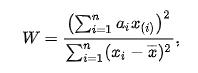# Shapiro-Wilks test and the order statistic

• I
Mayhem
TL;DR Summary
How to interpret the order statistic in the context of SW
Given the Shapiro-Wilk test value W:where I'm interested in the numerator. If my data is sorted in ascending order, my understanding is that $x_(i) = x_i$. Is that correct?

Last edited:

Mentor
Summary: How to interpret the order statistic in the context of SW

Given the Shapiro-Wilk test value W:
View attachment 302652
where I'm interested in the numerator. If my data is sorted in ascending order, my understanding is that $x_(i) = x_i$. Is that correct?
Sounds right to me. From the wiki page, https://en.wikipedia.org/wiki/Shapiro–Wilk_test:
##x_{(i)}## (with parentheses enclosing the subscript index i; not to be confused with ##x_i##) is the ith order statistic, i.e., the ith-smallest number in the sample

Mayhem
Staff Emeritus
Homework Helper
Gold Member
Mentor
The Wiki page seems to be saying explicitly that in general ##x_{(i)} \neq x_i##.
But if the elements of the sequence are already ordered from smallest to largest, then ##x_{(i)} = x_i##.

Staff Emeritus